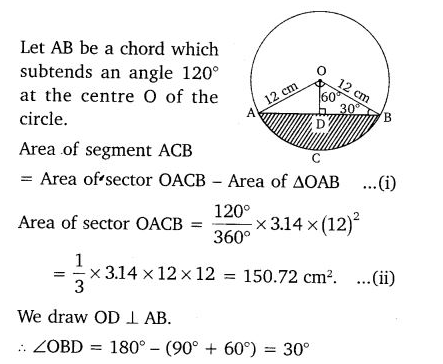• +91 9971497814
• info@interviewmaterial.com

# Areas Related to Circles Ex-12.2 Interview Questions Answers

### Related Subjects

Question 1 : Find the area of a sector of a circle withradius 6 cm if angle of the sector is 60°.

It is given that the angle of the sector is60°

We know that the area of sector = (θ/360°)×πr2

Area of the sector with angle 60° = (60°/360°)×πrcm2

= (36/6)π cm2

= 6×22/7 cm= 132/7 cm2

Question 2 :

Find the area of aquadrant of a circle whose circumference is 22 cm.

Circumference of the circle, C = 22 cm (given)

It should be noted that a quadrant of a circleis a sector which is making an angle of 90°.

Let the radius of the circle = r

As C = 2πr = 22,

R = 22/2π cm = 7/2 cm

Area of the quadrant = (θ/360°) × πr2

Here, θ = 90°

So, A = (90°/360°) × π rcm2

= (49/16) π cm2

= 77/8 cm2 = 9.6 cm2

Question 3 :

The length of theminute hand of a clock is 14 cm. Find the area swept by the minute hand in 5minutes.

Length of minute hand = radius of the clock(circle)

Radius (r) of the circle = 14 cm (given)

Angle swept by minute hand in 60 minutes =360°

So, the angle swept by the minute hand in 5minutes = 360° × 5/60 = 30°

We know,

Area of a sector = (θ/360°) × πr2

Now, area of the sector making an angle of 30°= (30°/360°) × πrcm2

= (1/12) × π142

= (49/3)×(22/7) cm2

= 154/3 cm2

Question 4 :

A chord of a circleof radius 10 cm subtends a right angle at the centre. Find the area of thecorresponding:

(i) minor segment

(ii) major sector.(Use π = 3.14)

Answer 4 :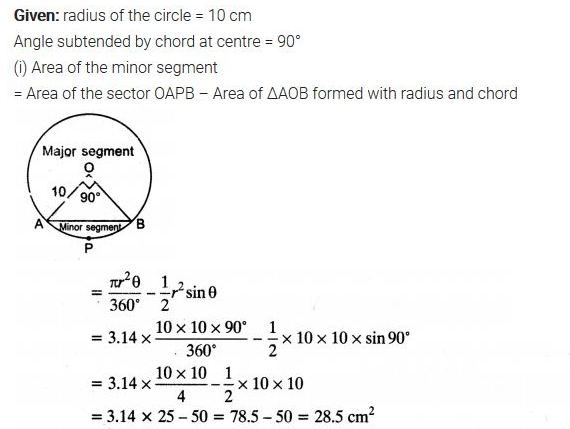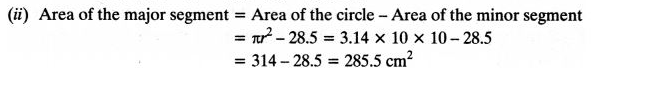Question 5 :

In a circle ofradius 21 cm, an arc subtends an angle of 60° at the centre.

Find:

(i) the length of the arc

(ii) area of the sector formed by the arc

(iii) area of the segment formed by the corresponding chord

Solution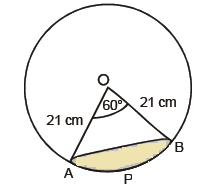Given,

θ = 60°

(i) Length of an arc = θ/360°×Circumference(2πr)

Length of an arc AB = (60°/360°)×2×(22/7)×21

= (1/6)×2×(22/7)×21

Or Arc AB Length = 22cm

(ii) It is given that the angle subtend by the arc = 60°

So, area of the sector making an angle of 60°= (60°/360°)×π rcm2

= 441/6×22/7 cm2

Or, the area of the sector formed by the arcAPB is 231 cm2

(iii) Area of segment APB = Area of sector OAPB – Area of ΔOAB

Since the two arms of the triangle are theradii of the circle and thus are equal, and one angle is 60°, ΔOAB is anequilateral triangle. So, its area will be √3/4×a2 sq. Units.

Area of segment APB = 231-(√3/4)×(OA)2

= 231-(√3/4)×212

Or, Area of segment APB = [231-(441×√3)/4] cm2

Question 6 :

A chord of a circleof radius 15 cm subtends an angle of 60° at the centre. Find the areas of thecorresponding minor and major segments of the circle. (Use π = 3.14 and √3 =1.73)

Answer 6 :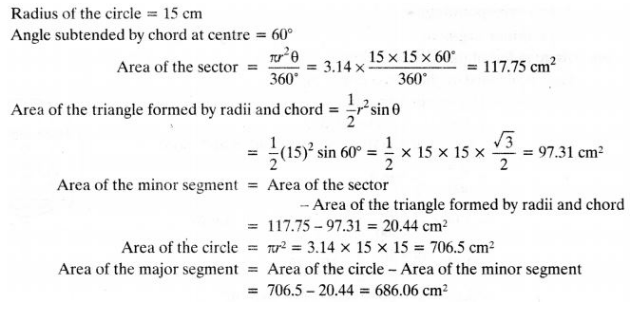Question 7 :

A chord of a circleof radius 12 cm subtends an angle of 120° at the centre. Find the area of thecorresponding segment of the circle. (Use π = 3.14 and √3 = 1.73)

Answer 7 :